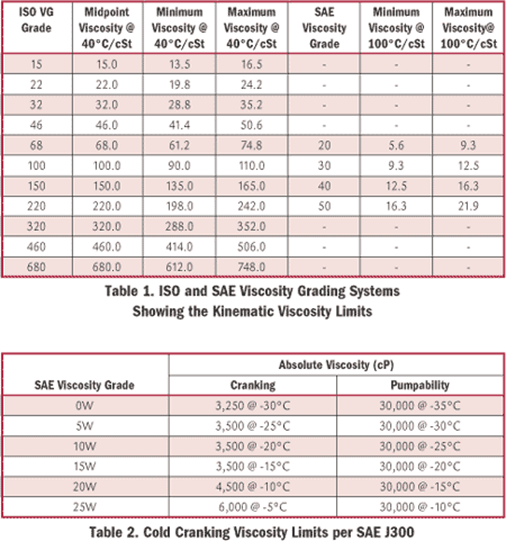## Viscosity definition and measure

Viscosity is a property of the fluid which opposes the relative motion between the two surfaces of the fluid that are moving at different velocities. In simple terms, viscosity means friction between the molecules of fluid.

## Viscosity definition and measure

The viscosity of a fluid is a measure of its resistance to gradual deformation by shear stress or tensile stress. It is a measure of the resistance of flow due to internal friction when one layer of fluid is caused to move in relationship to another layer.

Viscosity is a property of the fluid which opposes the relative motion between the two surfaces of the fluid that are moving at different velocities. In simple terms, viscosity means friction between the molecules of fluid. When the fluid is forced through a tube, the particles which compose the fluid generally move more quickly near the tube's axis and more slowly near its walls; therefore some stress (such as a pressure difference between the two ends of the tube) is needed to overcome the friction between particle layers to keep the fluid moving. For a given velocity pattern, the stress required is proportional to the fluid's viscosity.

Absolute Viscosity: The tangential force per unit area of two parallel planes at unit distance apart when the space between them is filled with a fluid and one plane moves with unit velocity in its own plane relative to the other. Also known as coefficient of viscosity.

Considering two parrallel plane of area A [m2] at distance y [m], one fixed and one moving under a Force F [N], the moving plane will move at velocity u [m/s].
In an ideal laminar flow, at low speed, the velocity variation would be linear and the shear stress τ will be proportional to distance.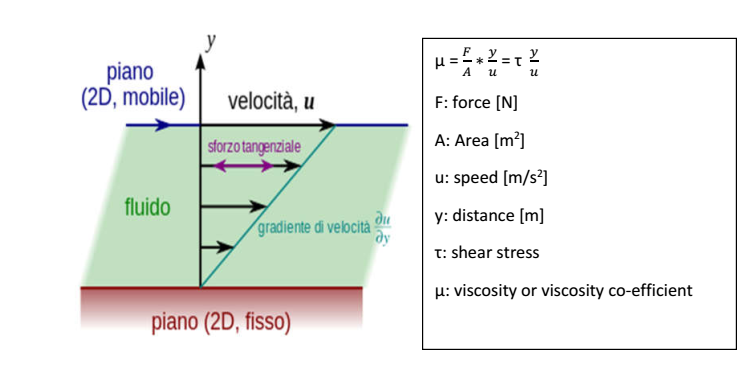Considering that the conditions described above are difficult to obtain a more realistic condition would be that the velocity would not increase linearly.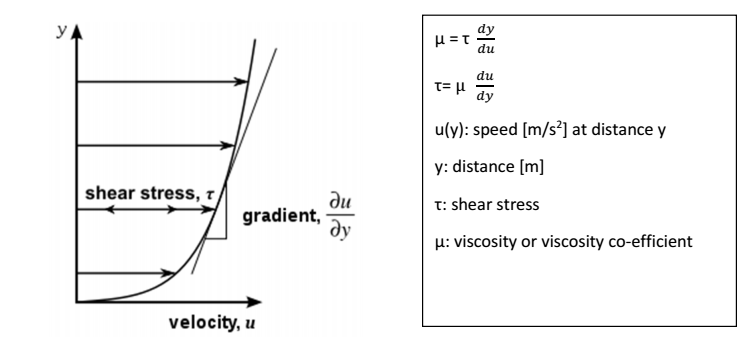The measuring unit of dynamic viscosity in SI is the pascal per second [Pa s], that is equal to a poiseuille [PI], often in lubricant industry the submultiple millipascal per second [mPa s] is used or in alternative the centipoise [cP] (CGS system) that is equal to 1 mPa s.
1 Pa s = 1 PI
1 P = 0,1 PI
1 cP = 1 mPI
In order to understand better the measuring unit used in viscosity:

The shear stress is defined as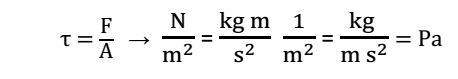where:
F: applied froce in Newton N
A: surface of the plane to which the force is applied in meter square m2

so we can consider: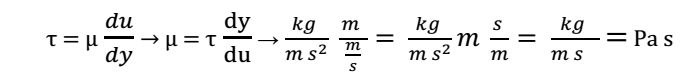where:
y: distance in meter m
u: velocity in meter per second m/s

Kinematic Viscosity: The absolute viscosity of a fluid divided by the density of the fluid. Also known as the coefficient of kinematic viscosity. The measuring unit of kinematic viscosity is meter square per second [m2/s], although in lubricants industry the submultiple millimeter square per second [mm2/s] is used, the mm2/s is equal to one centistokes (cSt) used in CGS system.
1 cSt = 1 mm2/s = 10−6 m2/s

In order to understand better the measuring unit: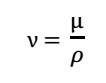where:
ρ: density  kg/m3
µ: dynamic viscosity  Pa s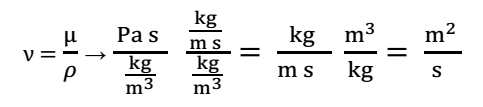Apparent Viscosity: The value obtained by applying the instrumental equations used in obtaining the viscosity of a Newtonian fluid to viscometer measurements of a non-Newtonian fluid.

Dilute Solution Viscosity: The viscosity of a dilute solution of a polymer, measured under prescribed conditions, is an indication of the molecular weight of the polymer and can be used to calculate the degree of polymerization.

Intrinsic Viscosity ([η]): The ratio of a solution’s specific viscosity to the concentration of the solute, extrapolated to zero concentration. Intrinsic viscosity reflects the capability of a polymer in solution to enhance the viscosity of the solution.

Equations:
Basic Viscosity
Shear Stress / Shear Rate = Viscosity

General
a = 9.801 m/s²

Dynamic Viscosity
1 poise = 1 g/cm·s = 0.1 N·s/m² = 0.1 Pa·s
1 cP = 1 mPa·s

SI Unit Conversion
1 Pascal = 1 Newton / m²

Kinematic Viscosity
1 Stoke = 1 cm²/s = 100 mm²/s
1 cSt = 1 mm²/s

Conversion from Rotational to Kinematic Viscosity
Poise / Density = Stoke
g/cm·s / g/cm3 = cm²/s

Conversion from Kinematic to Rotational Viscosity
Stoke * Density = Poise
cm²/s * g/cm3 = g/cm·s

Conversion from Kinematic to Saybolt Viscosity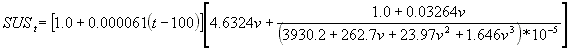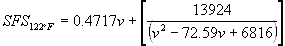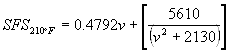Krebs Unit (for Thomas®-Stormer Viscometer)
Over the viscosity range of 200 to 2100 mPa·s (cP)
ln(KU) = 1.1187 + 0.8542*ln(0.1938v + 36) - 0.0443(ln(0.1938v +36))²

Over the viscosity range of 2100 to 5000 mPa·s (cP)
ln(KU) = 1.8118 + 0.596*ln(0.1938v + 36) - 0.0206(ln(0.1938v +36))²

Where KU is the viscosity in Krebs Units at 25°C
and v is the viscosity in mPa·s (cP) at 25°C

#### We sugest to download the following converter if you are intersted in having a powerful conversion simple software